## Doggie - A Cross-platform Library for Swift

Doggie is a foundational library for Apple's swift. It includes mathematics, accelerate, signal processing and graphic functions.

## Features

• atomic operation, async task and actor
• accelerate, signal processing, fourier and convolution
• complex number, point, vector and 2D/3D transformation
• bezier curve
• polynomial
• color and color space
• shape and boolean operation
``````let shape = try Shape(code: "M100 0c0-100-236.60 36.60-150 86.60S36.60-136.60-50-86.60 100 100 100 0z")

let region = ShapeRegion(shape, winding: .nonZero)
let ellipse = ShapeRegion(ellipseIn: shape.boundary)
``````
operation preview
`region.union(ellipse)`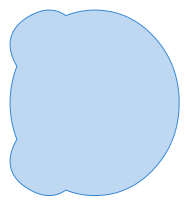`region.intersection(ellipse)`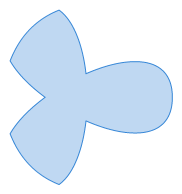`ellipse.subtracting(region)``region.subtracting(ellipse)`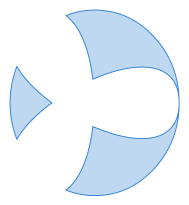`region.symmetricDifference(ellipse)`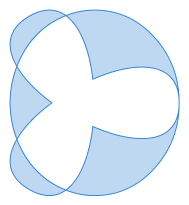• font
``````let collection = try FontCollection(data: fontFileData)

if let font = collection.first?.with(size: 64) {

let string = "Doggie\u{0301}".precomposedStringWithCanonicalMapping

let glyphs = font.glyphs(with: string.unicodeScalars)

var shape = Shape()

var outline = font.shape(forGlyph: glyph)
shape.append(contentsOf: outline.identity)
}

print(shape.encode())
}
``````
• image and graphics with 2D/3D drawing
``````
let context = ImageContext<ARGB32ColorPixel>(width: 100, height: 100, colorSpace: ColorSpace.sRGB)

let ellipse1 = Shape(ellipseIn: Rect(x: 10, y: 35, width: 55, height: 55))
let ellipse2 = Shape(ellipseIn: Rect(x: 35, y: 10, width: 55, height: 55))

context.draw(shape: ellipse1, winding: .nonZero, color: RGBColorModel(red: 247/255, green: 217/255, blue: 12/255))
context.stroke(shape: ellipse1, width: 1, cap: .round, join: .round, color: RGBColorModel())
context.draw(shape: ellipse2, winding: .nonZero, color: RGBColorModel(red: 234/255, green: 24/255, blue: 71/255))
context.stroke(shape: ellipse2, width: 1, cap: .round, join: .round, color: RGBColorModel())

let image: Image<ARGB32ColorPixel> = context.image
``````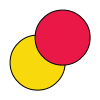• others
``````<svg width="200" height="200" viewBox="0 0 220 220"
xmlns="http://www.w3.org/2000/svg">
<filter id="displacementFilter">
<feTurbulence type="turbulence" baseFrequency="0.05"
numOctaves="2" result="turbulence"/>
<feDisplacementMap in2="turbulence" in="SourceGraphic"
scale="50" xChannelSelector="R" yChannelSelector="G"/>
</filter>

<circle cx="100" cy="100" r="100"
style="filter: url(#displacementFilter)"/>
</svg>
``````
``````let context = ImageContext<Gray16ColorPixel>(width: 220, height: 220, colorSpace: .default)

context.draw(ellipseIn: Rect(x: 0, y: 0, width: 200, height: 200), color: .black)

var image = context.image

let turbulence: Image<RGBA32ColorPixel> = SVGTurbulence(220, 220, .turbulence, nil, .identity, 0, 0.05, 2)

image = DisplacementMap(image, turbulence, 0, 1, 50)
``````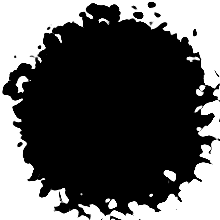## Playgrounds

Check out the Doggie.xcworkspace and build the project. It's suggested building in Release mode for the performance.

## Status

### ColorSpace

#### Format

• [x] ICC
• [x] CIEXYZ
• [x] CIELab
• [x] CIELuv
• [x] Calibrated Gray
• [x] Calibrated RGB

#### Rendering Intent

• [x] perceptual
• [ ] saturation
• [x] absolute colorimetric
• [x] relative colorimetric

• [x] bmp
• [ ] gif
• [ ] jpeg
• [ ] jpeg2000
• [x] png
• [x] tiff

### Font

#### Format

• [x] TrueType
• [x] TrueType/TTC
• [x] OpenType
• [x] OpenType/CFF
• [ ] OpenType/CFF2
• [x] WOFF
• [x] WOFF/CFF
• [ ] WOFF/CFF2
• [ ] WOFF2

#### SFNT Tables

• [x] POST
• [x] OS/2
• [x] MAXP
• [x] CMAP
• [x] NAME
• [x] HHEA
• [x] HMTX
• [x] VHEA
• [x] VMTX
• [x] LOCA
• [x] GLYF
• [x] SBIX
• [x] FEAT
• [ ] KERX
• [ ] MORX
• [x] Rearrangement
• [x] Contextual
• [x] Ligature
• [x] Noncontextual
• [ ] Insertion
• [ ] GSUB
• [ ] GPOS
• [ ] GDEF
• [x] CFF
• [ ] CFF2

#### CMAP

##### Platform

The table below lists the available platform/specific values in the order.

platform specific Description
0 max( <= 4 ) Unicode
3 10 Windows, Unicode UCS-4
3 1 Windows, Unicode BMP (UCS-2)
##### Format
• [x] Format 0
• [x] Format 4
• [x] Format 12
• [x] Format 14 (Unicode Variation Selectors)